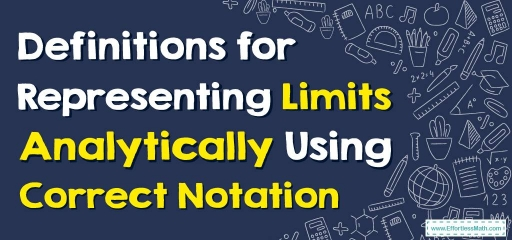# How to Define Limits Analytically Using Correct Notation?

In mathematics, a limit is defined as a value that a function approaches the output of a given input value. The following step-by-step guide helps you define limits analytically using correct notation.The Limits in maths are unique real numbers. Let’s consider a function with real value $$f$$ and the real number $$a$$, the limit is usually defined as the $$lim_{x\to a}{f(x)}=C$$. It is read as “the limit of $$f$$ of $$x$$, as $$x$$ approaches $$a$$ equals $$C$$“ . The “lim” shows the limit.

## A step-by-step guide to defining limits analytically using correct notation

Steps for Analytically Representing Limits Using the Correct Notation:

• The first step is to figure out what value $$x$$ is close to. This value will be $$a$$.
• The second step is to determine the function. $$f$$ is the name of the function $$(x)$$.
• The third step is to write the right notation for the limit analytically.

An Analytical Notation for Expressing Limits Analytically:

• A limit is an output $$(y)$$ value a function will approach while approaching a certain input $$(x)$$ value.
• When an input value approaches zero, the limit notation is used to show how close a function is to reaching its output value.

To show a limit analytically in the correct way, it should be written in this way:

### Defining Limits Analytically Using Correct Notation – Example 1:

Use correct notation to express the limit of $$f(x)=3x^2+2x-4$$ as $$x$$ approaches $$2$$.

First, identify $$a$$ value →  $$a=2$$

Then, identify $$f(x)$$ →  $$f(x)=3x^2+2x-4$$

Now, write the correct notation:

$$lim_{x\to 2}{(3x^2+2x-4)}=C$$

### Defining Limits Analytically Using Correct Notation – Example 2:

Use correct notation to express the limit of $$f(x)=x^4+10x+5$$ as $$x$$ approaches $$-3$$.

First, identify $$a$$ value →  $$a=-3$$

Then, identify $$f(x)$$ →  $$f(x)=x^4+10x+5$$

Now, write the correct notation:

$$lim_{x\to -3}{(x^4+10x+5)}=C$$

## Exercises for Defining Limits Analytically Using Correct Notation

1. Use correct notation to express the limit of $$f(x)=-3x-7x^2+20$$ as $$x$$ approaches $$12$$.
2. Use correct notation to express the limit of $$f(x)=-6x^3-5x+11$$ as $$x$$ approaches $$-6$$.
3. Use correct notation to express the limit of $$f(x)=5x^2+8x-3$$ as $$x$$ approaches $$-4$$.
4. Use correct notation to express the limit of $$f(x)=25x^5+22x^2-13$$ as $$x$$ approaches $$5$$.
1. $$\color{blue}{\lim_{x\to 12}(-3x-7x^2+20)=C}$$
2. $$\color{blue}{\lim_{x\to -6}(-6x^3-5x+11)=C}$$
3. $$\color{blue}{\lim_{x\to -4}(5x^2+8x-3)=C}$$
4. $$\color{blue}{\lim_{x\to 5}(25x^5+22x^2-13)=C}$$

### What people say about "How to Define Limits Analytically Using Correct Notation? - Effortless Math: We Help Students Learn to LOVE Mathematics"?

No one replied yet.

X
30% OFF

Limited time only!

Save Over 30%

SAVE $5 It was$16.99 now it is \$11.99